Well Hydraulics - 1

# Well Hydraulics - 1 - Notes | Study Foundation Engineering - Civil Engineering (CE)

 1 Crore+ students have signed up on EduRev. Have you?

Introduction

As established in the earlier lessons, an enormous amount of water is stored within the ground.  A small portion of that is in an unsaturated state but that below the water table, also called ground water, can be easily extracted for useful purpose, depending on the type and location within which the water exists.  It has been roughly estimated that of the global water resources, about 0.6 percent exists as ground water, out of which about half can be economically extracted with the present drilling technology.  In fact, the ground water is the largest source of fresh water on earth excluding the polar icecaps and glaciers.  Hence, ground water has been extracted on all regions of the world for different purposes and about nearly one fifth of all the water used in the world is obtained from ground water sources.

Evidence of extraction of water from dug wells has been found in the archeological remnants of Mohenjodaro. In many of the cities established during the medieval ages in India, the main source of water was dug wells, though people were dependent on surface water bodies like rivers or, lakes, or ponds, if that happened to be nearby. It is only during the past century that tube wells became popular as an easily operatable source of extraction of ground water.  Gradually with easy access to electricity deep tube wells have become a common source of water.  However, establishment of tube well extraction of water involves knowledge about the movement of water through the geological formations, which has been discussed in Lessons 2.5 and 2.6. Water may have to be extracted from formations ranging from sand, silt, clay, fractured rocks of different compositions etc., A well may be dug to extract water from a confined or an unconfined aquifer. Digging of more than one well in close vicinity affects each others’ yield as the drawdown of one influences the other.  This may be quantitatively estimated by theories of ground water flow applied to the radial flow of water to each well.  In this lesson, these theories are discussed, which would be helpful in designing such wells.

Imagine a farmer using a deep tube or a dug well as a source of water for irrigating his field.  The well may be fitted with a submergible pump or a centrifugal pump to draw out water and discharge at the head of a channel leading to the fields.  As long as the pump is not in operation, the water in the well remains at a steady at a level, at that of the water table (Figure 1).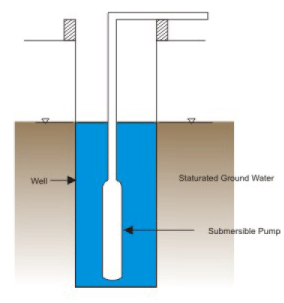Figure 1. Pump Not Started

When the pump is just started, it starts drawing out water from the well and the level of water in the well decreases. The water table surrounding the well also gets lowered (Figure 2).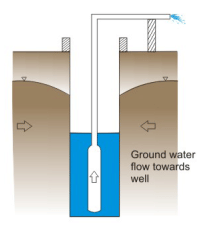Figure 2. Pump Just Started

The water table gets lowered and forms a conical depression much like that shown in Figure 3.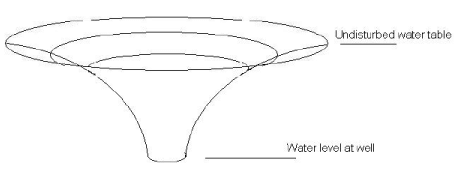FIGURE 3. Cone of depression

It may be observed that the surface of the water table, shaped now in the form of a cone, is steepest where it meets the well.  Farther away from the well, the surface is flatter and beyond a certain distance, called the radius of influence, the surface of the cone is almost as flat as the original water table.

As pumping continues (at the rated capacity of the pump), the water table gets lowered further until it becomes steady. At this position the water surface is called the draw down curve (Figure 4).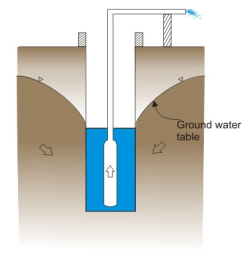In must be observed that the water that is being pumped up from the well is being replenished by water traveling through the saturated formation towards the well.  Further, if the capacity of the pump amount of water being in thrown from aware would be a lowered still.

Figures 1 to 4 depict a well that is drawing water from an unconfined aquifer. Corresponding figure of a steady state draw down curve in a confined aquifer would be as shown in Figure 5.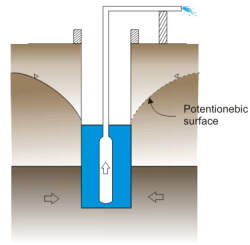Figure 5. Steady State Potentionetic Surface for Confined Agnifer

The following sections explain the mathematical relation between the water pumped and the location of the draw down.  It must be remembered that the flow towards the wells is actually taking place radially.  Hence, we shall be predominantly using the ground water flow equations using the cylindrical coordinates (r, θ, z, w) in contrast to the ones using Cartesian coordinates (x, y, z) as used in the previous lessons.

Steady and unsteady flow situations may further be classified as being confined or unconfined, depending on the relative positions of ground water conveying strata and the water table. The following sections describe each of these conditions individually.

Steady Flow to a Well: Confined Aquifer

Consider the case of a pumped well completely penetrating a confined aquifer (Figure 6). The corresponding steady state piezometric draw down surface is also shown for the assumed constant pumped discharge Q.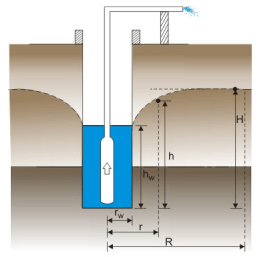FIG 6, Definition of terms

The well is assumed to have a redius rw and the radius of influence is thought to be R where the potentiometric surface is nearly equal to the original undisturbed value H, measured from a datum.  At the well, the depth of water is designated by hw, which is also measured from a common datum.  In general, at a certain radius r measured from the center of the well, the potentiometric surface stands at a height ‘h’ measured above the datum. The yield from the well Q may be expressed in terms of Darcy’s law as,

Q = K i A                         (1)

Where K is the coefficient of permeability of the formation, i is the hydraulic gradient that is, the slope of the potentiometric surface at the well bound and A is the surface area of the well through which the flow is converging into the well from the aquifer. Thus,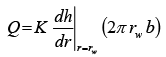(2)

In the above equation, b is the Thickness of the aquifer.

Naturally, the same amount of water also travels through the aquifer at a radial distance r from the center of the well. Thus, yield would also be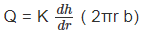(3)

The above expression is true if the aquifer thickness b is assumed to be constant throughout.  The above equations give us a value of the yield, Q, of the well but for that a measure of the gradient of the potentiometric surface is essential. This may be done by inserting a piezometer penetrating into the aquifer and noting the water level there (Figure 7).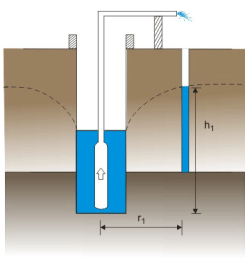Figure .7 Water Vise in Piezometer

Integrating (3) between the limits of rand r1, one obtains the following expressions: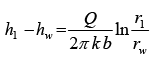(4)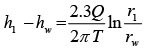(5)

Where T = Kb denotes the transmissibility of the aquifer.

This equation is known as equilibrium equation and can be used to determine variation of the potentiometric head radially outward from the well. The drawdown S at a radial distance from the well (Figure 8) is given by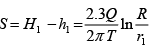(6)

Where H is the undisturbed initial potentimetric surface and R is the radius of influence. If the drawdown at distance r from the well known it is possible to work out T or K as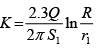(7)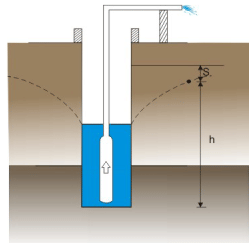Figure .8 Definition of Drawdown: S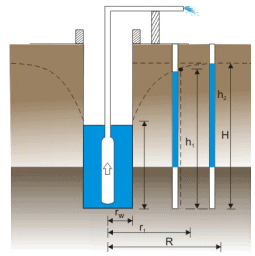Figure * 9 Definition of Terms

In case two piezometers are inserted near a well (Figure 9) and the piezometric head at these two places are given as h1 and h2, then the following expression is arrived at: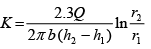(8)

This method of determining the permeability of an aquifer is known as Thiems method. Details about the method may be had from standard text books on ground water as the following:

Raghunath, H M (1998) Ground Water, Second Edition, New Age International Publishers.

The document Well Hydraulics - 1 - Notes | Study Foundation Engineering - Civil Engineering (CE) is a part of the Civil Engineering (CE) Course Foundation Engineering.
All you need of Civil Engineering (CE) at this link: Civil Engineering (CE)

## Foundation Engineering

18 videos|27 docs|20 tests
 Use Code STAYHOME200 and get INR 200 additional OFF

## Foundation Engineering

18 videos|27 docs|20 tests

Track your progress, build streaks, highlight & save important lessons and more!

,

,

,

,

,

,

,

,

,

,

,

,

,

,

,

,

,

,

,

,

,

;